# A 0.180 mole quantity of NiCl, is added to a liter of 1.20 M NH, solution....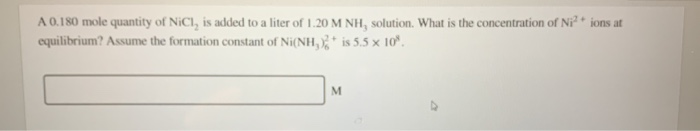A 0.180 mole quantity of NiCl, is added to a liter of 1.20 M NH, solution. What is the concentration of Ni?+ ions at equilibrium? Assume the formation constant of Ni(NH)2+ is 5.5 x 10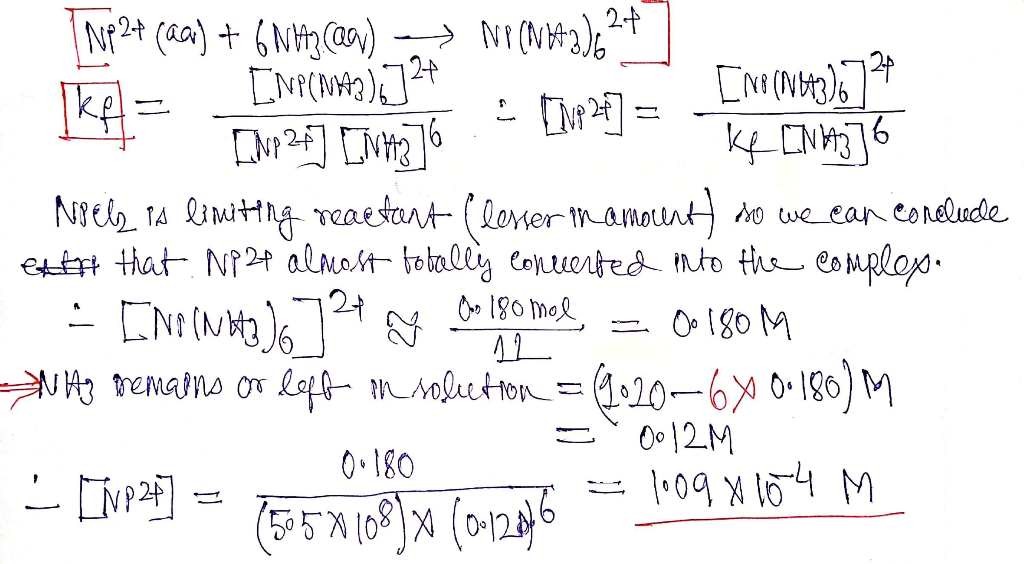#### Earn Coin

Coins can be redeemed for fabulous gifts.

Similar Homework Help Questions
• ### A 0.170 mole quantity of NiCl, is added to a liter of 1.20 M NH, solution....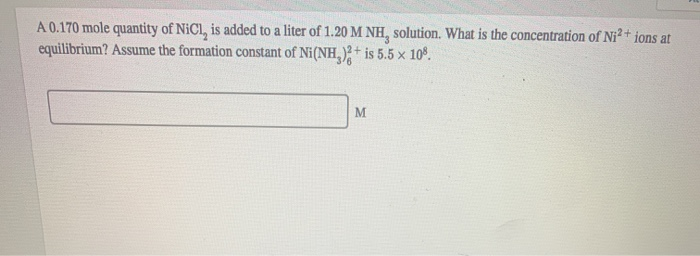A 0.170 mole quantity of NiCl, is added to a liter of 1.20 M NH, solution. What is the concentration of Nia + ions at equilibrium? Assume the formation constant of Ni(NH)2+ is 5.5 x 10%.

• ### A 0.170 mole quantity of NiCl, is added to a liter of 1.20 M NH2 solution....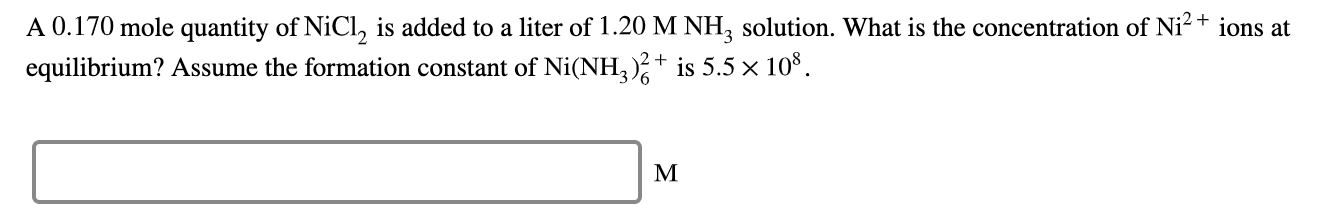A 0.170 mole quantity of NiCl, is added to a liter of 1.20 M NH2 solution. What is the concentration of Ni2+ ions at equilibrium? Assume the formation constant of Ni(NH2is 5.5 x 108 3 М

• ### P reedback Question 13 of 15 > ОА A 0.160 mole quantity of NiCl, is added...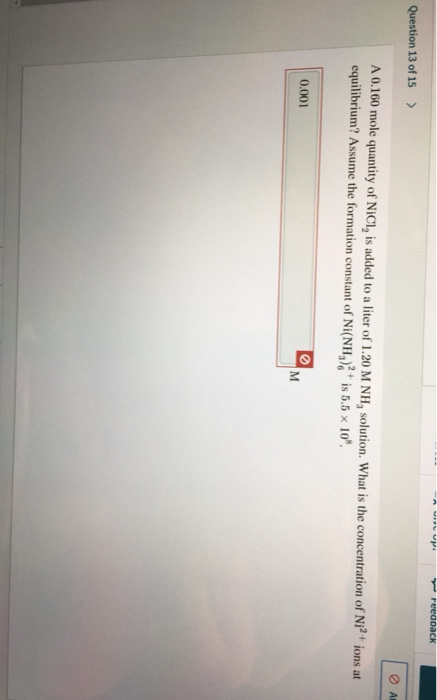P reedback Question 13 of 15 > ОА A 0.160 mole quantity of NiCl, is added to a liter of 1.20 M NH, solution. What is the concentration of Ni²+ ions at equilibrium? Assume the formation constant of Ni(NH) is 5.5 x 10° 0.001

• ### The formation constant* of [M(CN)2]-is 5.30 x 1018, where M is a generic metal. A 0.170...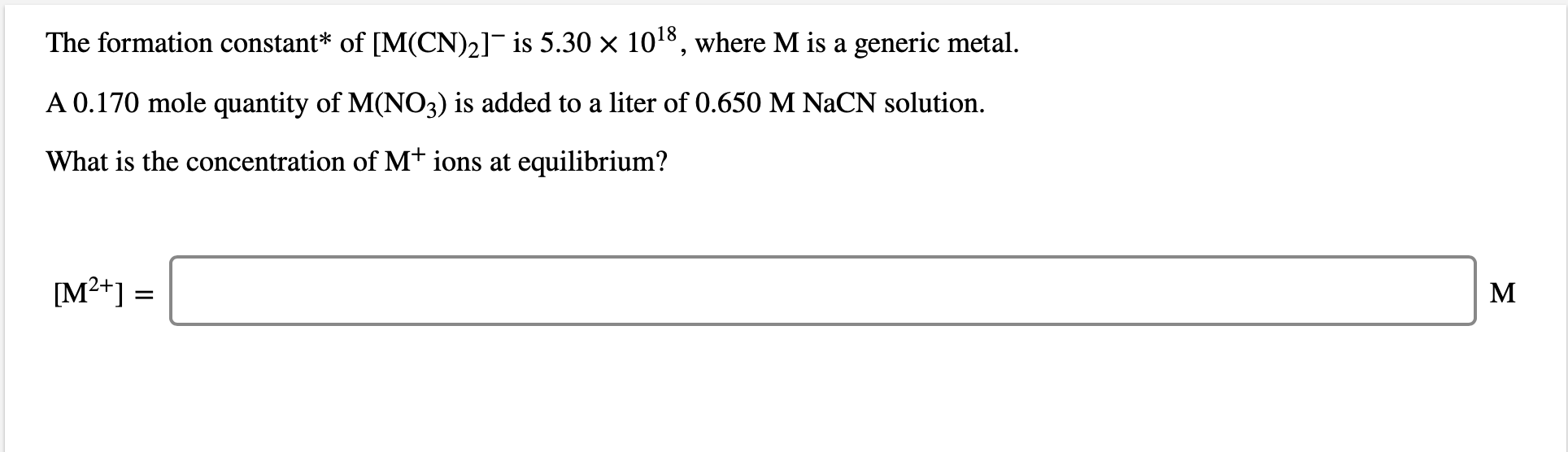The formation constant* of [M(CN)2]-is 5.30 x 1018, where M is a generic metal. A 0.170 mole quantity of M(NO3) is added to a liter of 0.650 M NaCN solution. What is the concentration of M+ ions at equilibrium? [M2+] =

• ### The formation constant* of [M(CN) 6 ]4− is 2.50 × 10 17 , where M is...

The formation constant* of [M(CN) 6 ]4− is 2.50 × 10 17 , where M is a generic metal. A 0.140 mole quantity of M(NO3)2 is added to a liter of 1.210 M NaCN solution. What is the concentration of M2+ ions at equilibrium?

• ### The formation constant of M(CN)614-is 2.50 x 10'7, where M is a generic metal. A 0.130...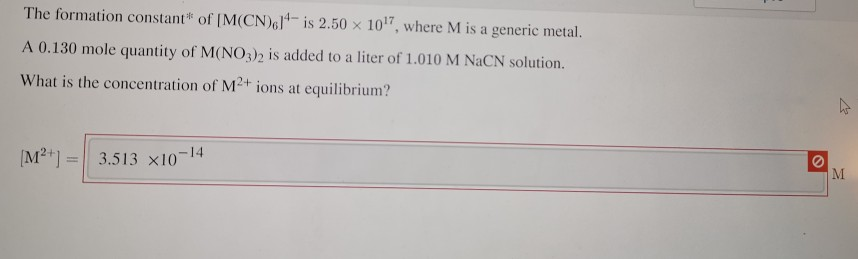The formation constant of M(CN)614-is 2.50 x 10'7, where M is a generic metal. A 0.130 mole quantity of M(NO3)2 is added to a liter of 1.010 M NaCN solution. What is the concentration of M2+ ions at equilibrium? [M²+] = 3.513 x10-14

• ### The formation constant* of [M(CN)2]– is 5.30 × 1018, where M is a generic metal. A...

The formation constant* of [M(CN)2]– is 5.30 × 1018, where M is a generic metal. A 0.170-mole quantity of M(NO3) is added to a liter of 0.540 M NaCN solution. What is the concentration of M ions at equilibrium?

• ### The formation constant* of [M(CN) 4 ]2− is 7.70×1016 , where M is a generic metal....

The formation constant* of [M(CN) 4 ]2− is 7.70×1016 , where M is a generic metal. A 0.130 mole quantity of M(NO3)2 is added to a liter of 0.870 M NaCN solution. What is the concentration of M2+ ions at equilibrium?

• ### The formation constant* of [M(CN)4]2– is 7.70 × 1016, where M is a generic metal. A...

The formation constant* of [M(CN)4]2– is 7.70 × 1016, where M is a generic metal. A 0.170-mole quantity of M(NO3)2 is added to a liter of 0.930 M NaCN solution. What is the concentration of M2 ions at equilibrium?

• ### The formation constant* of [M(CN)6]4– is 2.50 × 1017, where M is a generic metal. A...

The formation constant* of [M(CN)6]4– is 2.50 × 1017, where M is a generic metal. A 0.170-mole quantity of M(NO3)2 is added to a liter of 1.41 M NaCN solution. What is the concentration of M2 ions at equilibrium?